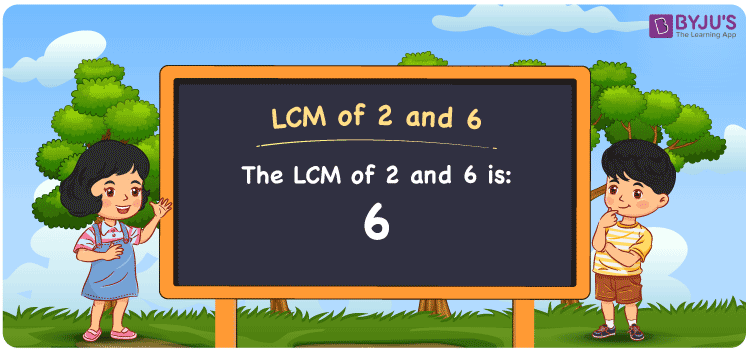Checkout JEE MAINS 2022 Question Paper Analysis : Checkout JEE MAINS 2022 Question Paper Analysis :

# LCM of 2 and 6

LCM of 2 and 6 is 6. In Mathematics, the LCM of given two numbers is the value evenly divisible by the numbers. Least common multiple of 2 and 6 can be obtained by knowing the common multiples of the two numbers. (2, 4, 6, 8, 10, 12, ….) and (6, 12, 18, 24, 30, 36,….) are the first set of multiples of the number 2 and 6. The LCM can be determined effortlessly using certain methods like prime factorisation, division method and by listing out the multiples.

## What is LCM of 2 and 6?

The answer to this question is 6. The LCM of 2 and 6 using various methods is shown in this article for your reference. The LCM of two non-zero integers, 2 and 6, is the smallest positive integer 6 which is divisible by both 2 and 6 with no remainder.## How to Find LCM of 2 and 6?

LCM of 2 and 6 can be found using three methods:

• Prime Factorisation
• Division method
• Listing the multiples

### LCM of 2 and 6 Using Prime Factorisation Method

The prime factorisation of 2 and 6, respectively, is given by:

2 = 2¹

6 = 2 x 3 = 2¹ x 3¹

LCM (2, 6) = 6

### LCM of 2 and 6 Using Division Method

We’ll divide the numbers (2, 6) by their prime factors to get the LCM of 2 and 6 using the division method (preferably common). The LCM of 2 and 6 is calculated by multiplying these divisors.

 2 2 6 3 1 3 x 1 1

No further division can be done.

Hence, LCM (2, 6) = 6

### LCM of 2 and 6 Using Listing the Multiples

To calculate the LCM of 2 and 6 by listing out the common multiples, list the multiples as shown below

 Multiples of 2 Multiples of 6 2 6 4 12 6 18 8 24 10 30

LCM (2, 6) = 6

## Video Lesson on Applications of LCM## LCM of 2 and 6 Solved Examples

If the product of two numbers is 12 and the GCD is 2, find the LCM.

Solution:

It is given that

Product of two numbers = 12

GCD = 2

We know that

LCM x GCD = Product of two numbers

LCM = Product/GCD

LCM = 12/2

LCM = 6

Hence, the LCM is 6.

## Frequently Asked Questions on LCM of 2 and 6

### Find the LCM of 2 and 6.

The LCM of 2 and 6 is 6.

We know that the smallest multiple which is exactly divisible by 2 and 6 has to be determined.

Multiples of 2 = 2, 4, 6, 8, …

Multiples of 6 = 6, 12, 18, 24, ….

The smallest multiple which is exactly divisible by 2 and 6 is 6.

### Which methods can be used to get the LCM of 2 and 6?

The methods that can be used to get the LCM of 2 and 6 are Prime Factorization Method, Division Method and Listing multiples.

### What is the GCF if the LCM of 2 and 6 is 6?

LCM x GCF = 2 x 6

Given

LCM of 2 and 6 = 12

6 x GCF = 12

GCF = 12/6 = 2